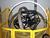Query Calculation Part of Select CriteriaTags:
iSeries Navigator
Query Calculation
SQL
SQL scripts
This query works great except I need to add one thing. There are two district codes that need to be included in the query. These codes just need the MC_Tax_Amount field totaled - no dividing by two. How do I add this to my query? Thank you,

SELECT "T16.CPRP".CP_DISTRICT_CODE, SUM("T16.CMCL".MC_TAX_AMOUNT/2) AS TOWN , SUM("T16.CMCL".MC_TAX_AMOUNT/2) AS COUNTY

FROM QS36F."T16.CPRP" AS "T16.CPRP", QS36F."T16.CMCL" AS "T16.CMCL"

WHERE "T16.CPRP".CP_YEAR = "T16.CMCL".MC_OCC_YEAR AND "T16.CPRP".CP_DISTRICT_CODE BETWEEN 1159 AND 1561 AND "T16.CPRP".CP_PROPERTY_NO = "T16.CMCL".MC_OCC_PARCEL_NR AND "T16.CPRP".CP_ROLL_TYPE = "T16.CMCL".MC_OCC_ROLL AND "T16.CMCL".MC_RECEIPT_DATE BETWEEN 20091201 AND 20091231

GROUP BY "T16.CPRP".CP_DISTRICT_CODE;

Software/Hardware used:
Iseries Navigator Run SQL Script
Related Questions

Thanks. We'll let you know when a new response is added.

Try something like this:
<pre>SELECT “T16.CPRP”.CP_DISTRICT_CODE,
CASE “T16.CPRP”.CP_DISTRICT_CODE
WHEN ‘xx’ THEN SUM(“T16.CMCL”.MC_TAX_AMOUNT) AS TOWN
WHEN ‘yy’ THEN SUM(“T16.CMCL”.MC_TAX_AMOUNT) AS TOWN
ELSE SUM(“T16.CMCL”.MC_TAX_AMOUNT/2) AS TOWN
END,
SUM(“T16.CMCL”.MC_TAX_AMOUNT/2) AS COUNTY</pre>

(Replace ‘xx’ and ‘yy’ with the special district-codes which shall not be divided. And let the rest of your code remain.

Happy coding

—————————————

It could be done in different ways depending on the database.

One option could be the use of a CASE expression.

Something like this (in this example, 1 and 2 are the codes that don’t need the MC_Tax_Amount divided by 2) :

<pre>SELECT “T16.CPRP”.CP_DISTRICT_CODE,
SUM(“T16.CMCL”.MC_TAX_AMOUNT/CASE “T16.CPRP”.CP_DISTRICT_CODE
WHEN 1 THEN 1
WHEN 2 THEN 1
ELSE 2 END) AS TOWN ,

…</pre>

In oracle, the DECODE function would simplify this.

-CarlosDL

—————————————-

Discuss This Question: 7 Replies

There was an error processing your information. Please try again later.
Thanks. We'll let you know when a new response is added.
Send me notifications when members answer or reply to this question.
• When I run the query it stops on "AS Town" states keyword not expected here. SELECT "T16.CPRP".CP_DISTRICT_CODE, CASE "T16.CPRP".CP_DISTRICT_CODE WHEN '"T16.CPRP".CP_DISTRICT_CODE BETWEEN 1159 AND 1561' THEN SUM("T16.CMCL".MC_TAX_AMOUNT/2) AS TOWN WHEN '"T16.CPRP".CP_DISTRICT_CODE BETWEEN 1159 AND 1561' THEN SUM("T16.CMCL".MC_TAX_AMOUNT/2) AS COUNTY ELSE SUM("T16.CMCL".MC_TAX_AMOUNT/2) AS TOWN END, SUM("T16.CMCL".MC_TAX_AMOUNT/2) AS COUNTY FROM QS36F."T16.CPRP" AS "T16.CPRP", QS36F."T16.CMCL" AS "T16.CMCL" WHERE "T16.CPRP".CP_YEAR = "T16.CMCL".MC_OCC_YEAR AND "T16.CPRP".CP_DISTRICT_CODE BETWEEN 1159 AND 1561 AND "T16.CPRP".CP_PROPERTY_NO = "T16.CMCL".MC_OCC_PARCEL_NR AND "T16.CPRP".CP_ROLL_TYPE = "T16.CMCL".MC_OCC_ROLL AND "T16.CMCL".MC_RECEIPT_DATE BETWEEN 20091201 AND 20091231 GROUP BY "T16.CPRP".CP_DISTRICT_CODE; Thanks again,
report
•I think you will have to put the alias (AS TOWN) at the end of the case expression (not after every option)
CASE <something>
WHEN ...
WHEN ...
ELSE
END AS COUNTY
Also, you could try the second suggestion (mine ;-) ) which I think is a little simpler
report
• I have modified the query - it doesn't like the "=" sign - it stops there and says Token was not valid SELECT "T16.CPRP".CP_DISTRICT_CODE, SUM(("T16.CMCL".MC_TAX_AMOUNT/CASE "T16.CPRP".CP_DISTRICT_CODE WHEN "T16.CPRP".CP_DISTRICT_CODE = 13 or 14 THEN SUM("T16.CMCL".MC_TAX_AMOUNT) as Town ELSE SUM("T16.CMCL".MC_TAX_AMOUNT/2) as County END), Thanks for the help.
report
•Please look at the examples provided. The correct syntax for a simple case is:
Simple CASE expression:
CASE input_expression
WHEN when_expression THEN result_expression [ ...n ]
[ ELSE else_result_expression ]
END
There is also a 'searched' case, whose syntax is:
CASE
WHEN Boolean_expression THEN result_expression [ ...n ]
[ ELSE else_result_expression ]
END
So, if you want to use a simple case, your code would look like this:
SELECT “T16.CPRP”.CP_DISTRICT_CODE,
SUM(”T16.CMCL”.MC_TAX_AMOUNT/CASE “T16.CPRP”.CP_DISTRICT_CODE
WHEN  13 THEN 1
WHEN  14 THEN 1
ELSE 2
END) as County,
If you want to use a searched case, then it would look like this:
SELECT “T16.CPRP”.CP_DISTRICT_CODE,
SUM(”T16.CMCL”.MC_TAX_AMOUNT/CASE
WHEN “T16.CPRP”.CP_DISTRICT_CODE in (13,14) THEN 1
ELSE 2
END) as County,
P.s. I'm not sure every database platform support both of these forms of CASE expressions.
report
• I'm guessing the "then 1" means perform the first calculation how does it know what the "else 2" means? Should I add the second sum statement somewhere? Before or after the end? Thanks!
report
•The query is using the CASE expression as the divisor, so, if the district code is 13 or 14, we want it to return 1 (mc_tax_amount/1 = mc_tax_amount), otherwise we want it to return 2. For example, when the district code is 13 or 14, this will be executed: SUM(”T16.CMCL”.MC_TAX_AMOUNT/1) Because the case is returning 1 Otherwise, this will be executed: SUM(”T16.CMCL”.MC_TAX_AMOUNT/2) Because the case is returning 2 If you want to avoid the unnecessary division by 1 when the district code is 13 or 14, you could do something similar to the example provided by DanTheDane.
SELECT “T16.CPRP”.CP_DISTRICT_CODE,
CASE
WHEN “T16.CPRP”.CP_DISTRICT_CODE in (13,14) THEN SUM(”T16.CMCL”.MC_TAX_AMOUNT)
ELSE SUM(”T16.CMCL”.MC_TAX_AMOUNT/2)
END) as County,
report
•and if CP_DISTRICT_CODE is a character type you may need quotes around the values in ('13','14')
report